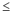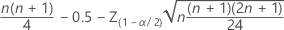# Calculate the estimated median and confidence interval for the 1-sample Wilcoxon test

## Estimated Median

Suppose the following data are in C1, and C2 and C3 are empty:

24 12 24 19 12 21 23 11 17 19 23 14 23 15 14 6

1. Calculate W(i) = (Xi + Xj)/2 = all pairwise averages for ij.
1. Choose Stat > Nonparametrics > Pairwise Averages.
2. In Variable, enter C1.
3. In Store averages in, type C2. Click OK.
2. Calculate the estimated median
1. Choose Stat > Basic Statistics > Store Descriptive Statistics.
2. In Variables, enter C2.
3. Click Statistics and check only Median.
4. Click OK in each dialog box
The resulting stored value (17.5) is the Estimated Median for the 1-Sample Wilcoxon test.

## Confidence Interval

Suppose the following data are in C1, and C2 and C3 are empty:

24 12 24 19 12 21 23 11 17 19 23 14 23 15 14 6

1. Calculate W(i) = (Xi + Xj)/2 = all pairwise averages for ij.
1. Select Stat > Nonparametrics > Pairwise Averages.
2. In Variable, enter C1.
3. In Store averages in, type C2. Click OK.
2. Put the pairwise averages in numerical order from lowest to highest.
1. Select Data > Sort.
2. In Columns to sort by, enter C2 under Column.
3. In Columns to sort select Specified columns.
4. In Columns, enter C2.
5. Under Storage location for the sorted columns, select In the original columns. Click OK.
The sorted pairwise averages are in C2.
3. To get the endpoints of the (1-α)*100% confidence interval, first solve for Z(1-α/2). For a 95% confidence interval:
1. Select Calc > Probability Distributions > Normal.
2. Select Inverse cumulative probability.
3. Select Input constant and enter 0.975. Click OK.
4. Next, calculate d, which is approximately1. Select Calc > Calculator.
2. In Store result in variable, enter C3.
3. in Expression, enter 16*17/4-.05-1.96*sqrt(16*17*33/24). Click OK.
5. Using the notation above, the lower endpoint of the confidence interval is W(d+1) and the upper endpoint is W(nw-d), where nw is the number of pairwise averages.

W(d+1) = W(31). The 31st pairwise average in C2 is 14.5.

W(nw-d) = W(136-30) = W(106). The 106th pairwise average in C2 is 21.

### Wilcoxon Signed Rank CI: C1

Method η: median of C1
Descriptive Statistics Achieved Sample N Median CI for η Confidence C1 16 17.5 (14.5, 21) 94.75%
###### Note

Because d (which approximates the Wilcoxon test statistic) is positive, it will seldom be possible to achieve the specified confidence. The procedure prints the closest value, which is computed using a normal approximation with a continuity correction.

By using this site you agree to the use of cookies for analytics and personalized content.  Read our policy